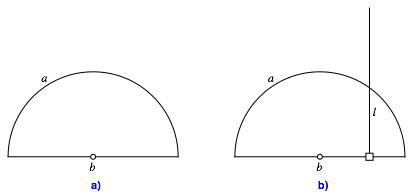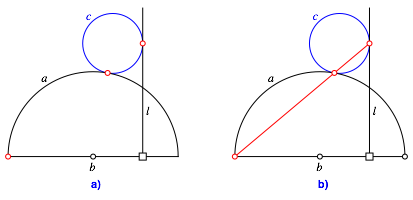Hans Walser, 

Collinear points

# 1     Three collinear points

Let a be a half circle with base b and l a line orthogonal to b (Fig. 1).Fig. 1: Half circle and orthogonal line

We draw a circle c of arbitrary radius touching the half circle a and the line l (Fig. 2a).Fig. 2: A circle and three collinear points

Now the two touching points and one endpoint of the half circle are collinear (Fig. 2b).

# 2     Proof

The figure 3 gives a proof without words.Fig. 3: Proof without words